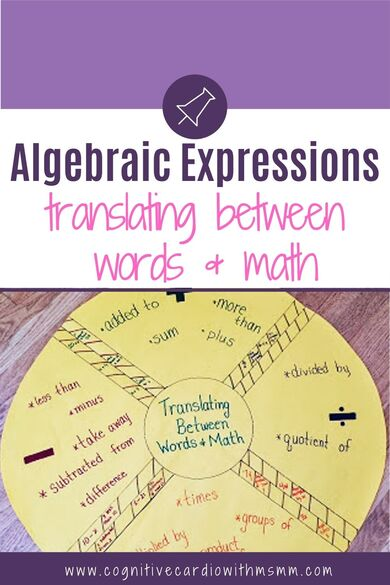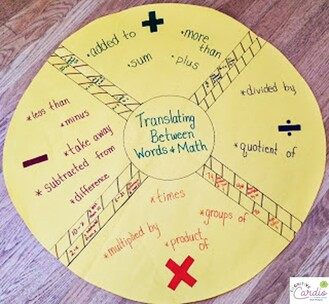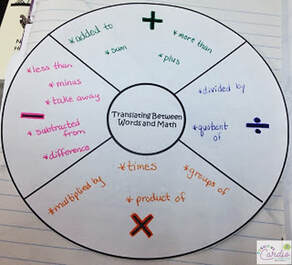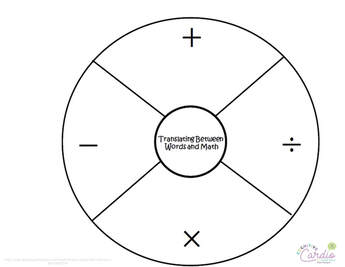# Translating Words into Algebraic Expressions

## Algebraic Expressions in 6th Grade MathTranslating between words and math in 6th grade – sometimes this can be easy, but some phrases can definitely be tricky!

By the time we start ‘officially’ translating between words and algebraic expressions, we’ve already done some translating to numerical expressions through our daily spiral review.

To connect translating words to numerical expressions and translating between words and algebraic expressions, we take some more time to translate between words and numerical expressions. We use an organizer to list the key words that typically signal the different operations. (I use the same type of circle organizer I use for my “daily spiral review” templates.)

We typically include the following math terms and phrases, as you can see in the organizer:

• sum
• plus
• **more than

Subtraction

• minus
• take away
• subtracted from
• difference
• **less than

Multiplication

• times
• multiplied by
• groups of
• product of

Division

• divided by
• quotient of

## Translating Words to Expressions – Tricky PhrasesThe phrases I starred in the list above (‘less than’ and ‘more than’) are always tricky for some students.
6th grade math students often want to use the ‘less than’ or ‘greater than’ signs when they translate a phrase like ‘ten more than seven.’

To help students clarify the idea that ‘more than’ means ‘addition’ and ‘less than’ means ‘subtraction’, I do the following:

• Emphasize that ‘>’ and ‘<‘ are read as ‘IS less than’ and ‘IS more than’
• Ask students to phrase ‘ten more than seven’ as a question – “What is 10 more than 7?” They are able answer this as 17, which helps them  realize that they have added for this phrase.​
• Substitute ‘subtracted from’ for ‘less than’: ‘8 less than 20’ can become ‘8 subtracted from 20.’
• Discuss the idea that for ‘less than’ and ‘more than,’ the number that’s being added or subtracted will be last in the phrase, like 7 + 10.

When we move to algebraic expressions like ‘ten more than x” we talk about substituting a number in place of the x, before they decide on the algebraic expression to write.

## Graphic Organizer for Translating Between Words and MathThis organizer is pretty simple. The first year I used it with my 6th graders, I gave students the option to use the printed organizer or draw their own. While most used the printed version, creating their own wasn’t too difficult.
After adding the operation terms, students added these to their interactive math notebooks (if they drew their own, they drew right in their notebooks.)

## Translating from Words to Algebraic ExpressionsWhen we make the transition to translating between words and algebraic expressions, I typically:

1. Emphasize the importance of paying attention to the order of operations.
2. Encourage students to underline the operation terms and then look at what numbers/terms are around them.

For example, for the phrase, ’20 divided by the sum of a number and 3′:

• Students might start by writing 20 ÷ x + 3
• Asking them to follow the order of operations should help them realize the algebraic expression they wrote really indicates ‘3 added to the quotient of 20 and a number‘
• Instead they need to indicate that 20 is being divided by a sum. That sum should go in parentheses to indicate that adding happens before division in this phrase: 20  ÷ (x + 3).
• If they underline the operation terms, if can help make things a little more clear: 20 divided by the sum; sum of a number and 3

Similarly, when students covert an algebraic expression to words, they need to be sure to indicate what should happen first in that expression.
For example, for the algebraic expression, (x + 8) ÷ 5:

• Students might start by writing ‘a number plus 8 divided by 5.’ But, following the order of operations for their phrase, 8 divided by 5 would be first.
• Instead, students need to indicate that the sum is being divided by 5, like ‘divide the sum of a number and 8 by 5.’

Translating between words and algebraic expressions can be tricky, but using strategies like substituting, underlining and following the order of operations definitely helps!

What strategies do you use to teach translating between words and algebraic expressions?

## EllieWelcome to Cognitive Cardio Math! I’m Ellie, a wife, mom, grandma, and dog ‘mom,’ and I’ve spent just about my whole life in school! With nearly 30 years in education, I’ve taught:

• All subject areas in 4th and 5th grades
• Math, ELA, and science in 6th grade (middle school)

I’ve been creating resources for teachers since 2012 and have worked in the elearning industry for about five years as well!